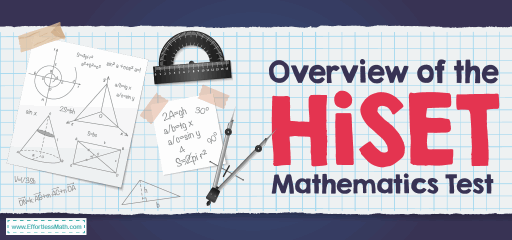# Overview of the HiSET Mathematics TestThe High School Equivalency Test as known as HiSET is used to assess whether a student has the academic skills that are equivalent to a typical high school graduate. The HiSET exam is an alternative to the GED exam. Passing the HiSET test allows students to obtain a high school equivalency credential issued by their state.

The HiSET test consists of 5 subtests:

• Mathematics
• Science
• Language Arts – Writing
• Social Studies

The HiSET math subtest is a measure of students’ understanding of basic math knowledge and skills, such as problem-solving fundamental concepts and reasoning skills. The HiSET math test will have a total of about 50 questions. All questions will be in the form of multiple-choice. You are expected to complete these questions in an hour and a half (90 minutes).

## What does cover in the HiSET Mathematics test?

The math part of the HiSET exam includes four distinct knowledge groups, with each test question falling under one of them. These categories are as follows:

### 1-Algebraic Concepts:

Algebraic concepts make up the largest number of test questions, as they make up 45% of the full test content. As the name implies, the questions in this subgroup deal mainly with the strategies and ideas taught in the average Algebra class, including graphing, expressions, equations, and similar elements.

### 2- Numbers and Operations on Numbers:

Numbers and operations on numbers account for 19% of the test questions. The questions in this category require you to work with numbers in a variety of ways, from rounding them up to converting them to another format.

## The Absolute Best Book to Ace the HiSET Math Test

### 3-Data Analysis, Probability, and Statistics:

Data analysis, probability, and statistics are some of the smallest categories, accounting for 18% of the test content. This category consists of similar problem-solving strategies, which will require you to interpret math data.

### 4-Measurement and Geometry:

Measurement and geometry also make up 18% of the test questions. This final group will ask you to evaluate the shapes using the information given to calculate their size and similar qualities.

In addition to these categories of knowledge, test questions are organized under what is known as Process Categories – or, more specifically, the strategies you should use to answer test questions. These strategies require you to synthesize data and solving problems, analyzing and interpreting information and understanding mathematical concepts and methods.

## Do you receive a formula sheet on the HiSET Mathematics test?

You will receive a reference table full of formulas related to the test content. This formula sheet will not be comprehensive. Likewise, it is better to memorize some basic formulas before participating in the mathematics subsection. The formulas that will not be presented to you in this test include the quadratic formula, the Pythagorean theorem, and distance-rate-time.

## Can you use a calculator on the HiSET Mathematics test?

Unlike any other part of HiSET, you can use the option to use a calculator throughout the whole duration of the section, although it is optional. We recommend checking which type of calculator is allowed on the chosen testing site. Some states have special conditions that calculators can be used during the test period. This step is to help you prepare, as it is forbidden to bring the calculator from home to the test site. Instead, you may want to ask for a calculator, which may be scientific or basic, during the test period.

## Is the HiSET Mathematics test hard?

The HiSET Math test, like any other test, can be challenging. However, if you are prepared enough to answer the HiSET Math questions, you will have no problem passing this test.

## How is the HiSET Mathematics test scored?

Most states use the HiSET passing criteria, which means you must pass the HiSET test. You need to get at least 8 out of 20 points in all five subtests. Get a total scaled score on all five HiSET subtests of at least 45 out of 100.

## The Best Books to Ace the HiSET Math Test

### What people say about "Overview of the HiSET Mathematics Test - Effortless Math: We Help Students Learn to LOVE Mathematics"?

No one replied yet.

X
52% OFF

Limited time only!

Save Over 52%

SAVE \$40

It was \$76.99 now it is \$36.99